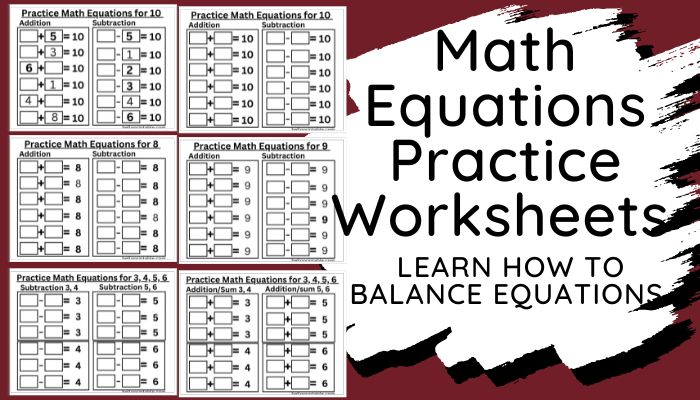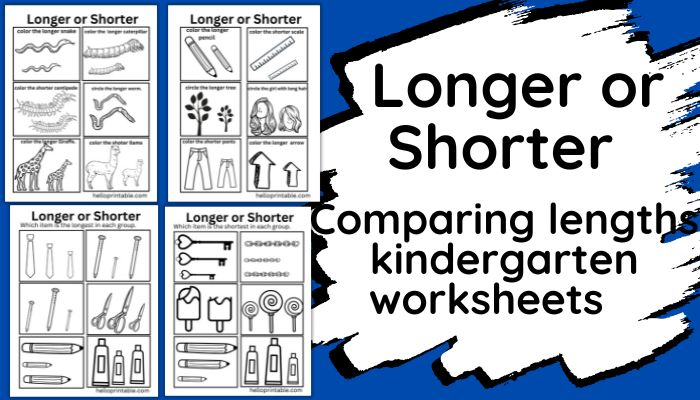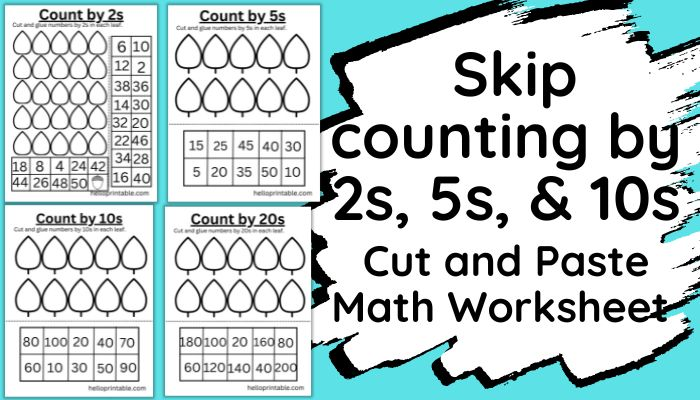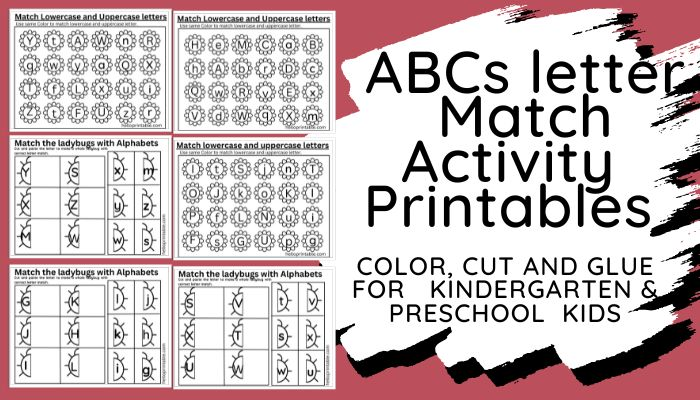## Learning Math Equations -Practice Worksheets

An equation is a mathematical expression with two sides separated by an equal sign. 2 + 4 = 6 is an example of an equation. The left-hand side of the equation is 2 + 4 before the equal to (=) sign – and the right-hand side is 6 after the equal to (=) sign....## Longer or shorter? Compare lengths worksheets

Learning about lengths is an important concept that is introduced at different levels in preschool and kindergarten classes. Comparing lengths, heights and widths is part of early introductory lessons to under different types of lengths. It instills the ability to...## Counting by 2s, 5s,10s Cut & Glue Worksheets

Counting by 2s, 5s, 10s, and bigger numbers is a great mental math exercise for young kids. Keep your kindergarteners, grade 1 and grade 2 students busy in this counting 2s, 5s, 10s, and 20s printable worksheets. These worksheets involve cut-and-paste gluing...## Alphabet Matching Activity for Preschool

In this post you will find free printable matching alphabet worksheets suitable for preschool and kindergarten kids. This printable bundle helps students recognize and differentiate between uppercase and lowercase letters of the ABC. The students learn capital letters...